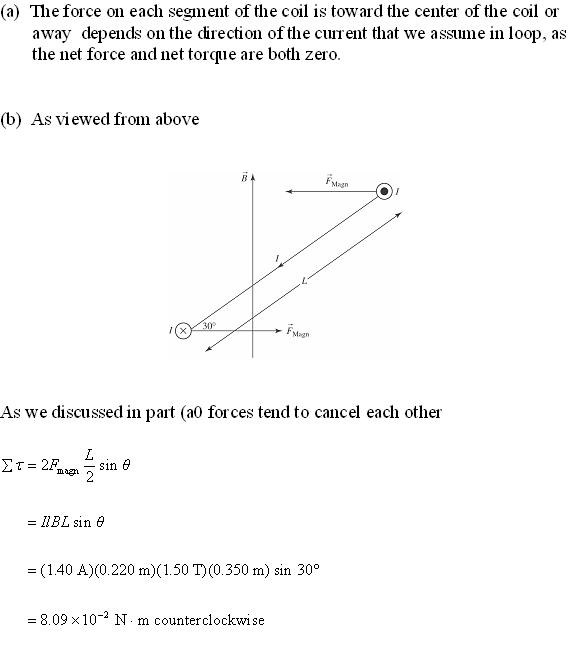preprekomW

2020-12-03

A rectangular coil of wire, 22cm by 35 cm and carrying a current of 1.4A, is oriented with the plane of its loop perpendicular to a uniform 1.5T magnetic field.
a) calculate the net force and torque which the magnetic field exerts on the coil.
b)the coil is rotated through a 30 degree angle about the y axis, the left side coming out of the plane. Calculate the net force and torque which the magnetic field now exerts on the coilExpert.

Do you have a similar question?